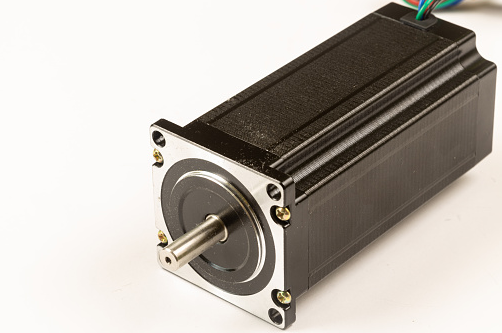## Induction Motor

A 2 pole induction motor operates with a 0.10 slip, and a line voltage frequency of 60 Hz. What is the motor shaft's rotational speed (rpm)?Hint
The synchronous speed for ac motors is:
$$n_s=120*f/p$$$where $$f$$ is the line voltage frequency in Hz, and $$p$$ is the number of poles. Hint 2 The slip for an induction motor is: $$slip=\frac{(n_s-n)}{n_s}$$$
where $$n$$ is the rotational speed.
The synchronous speed for ac motors is:
$$n_s=120*f/p$$$where $$f$$ is the line voltage frequency in Hz, and $$p$$ is the number of poles. For a 2 pole motor operating on 60 Hz voltage: $$n_s=120*60/2=3,600\:rpm$$$
The slip for an induction motor is:
$$slip=\frac{(n_s-n)}{n_s}$$$where $$n$$ is the rotational speed. $$0.10=\frac{(3,600rpm-n)}{3,600rpm}$$$
Solving for $$n$$ :
$$n=3,600rpm-0.1(3,600rpm)=3,600rpm-360rpm=3,240\:rpm$$\$
3,240 rpm